Courses
Courses for Kids
Free study material
Free LIVE classes
More

# Analogue Clock for Kids to Learn Time## An Introduction to Clocks

I am sure you might have seen different types of clocks. Some might have numbers written on them, some might have hour, minute and second hands on them. There are basically two types of clocks - analogue and digital. Let’s first know what a clock is before going into the analogue clock.

A clock is a device that measures and represents time. Some clocks show only time, whereas others show temperature, date, weather, etc. Also, some clocks have a stopwatch and some have alarms.

The two clocks that are widely used are analogue clock and digital clock. Both the clocks have different ways to represent time. An analogue clock is a clock that shows time with two or three moving hands that move in a circular motion. On the other hand, a digital clock is a clock that simply uses numbers to represent time.

Here, we will discuss analogue clocks in detail.

## What are Analogue Clocks?

A clock is known as an analogue clock when it has moving hands and is usually (hours) marked from 1 to 12 to show you the time of the day.

In some analogue clocks, hours are marked with Roman numerals (such as I, II, III, IV, etc.), while in others, there are no numbers marked in the clock.

## What are the Hands of Analogue Clock?

As discussed above, analogue clocks commonly have two or three hands. If an analogue clock has two hands, then there is a:

• Larger hand, known as a minute hand.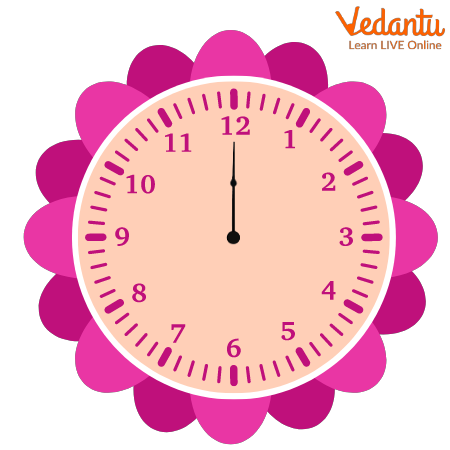Image: Minute hand

• Smaller hand, known as an hour hand.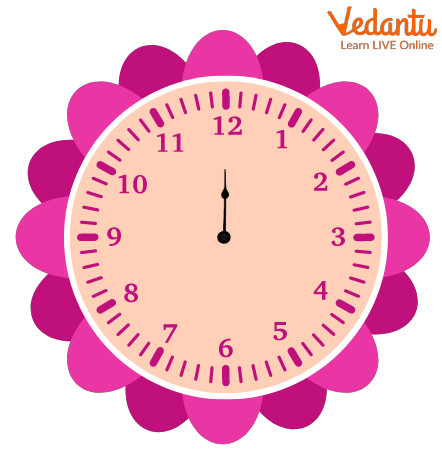Image: Hour hand

A clock with three hands has a second hand. The second hand is as long as the hour hand but much thinner.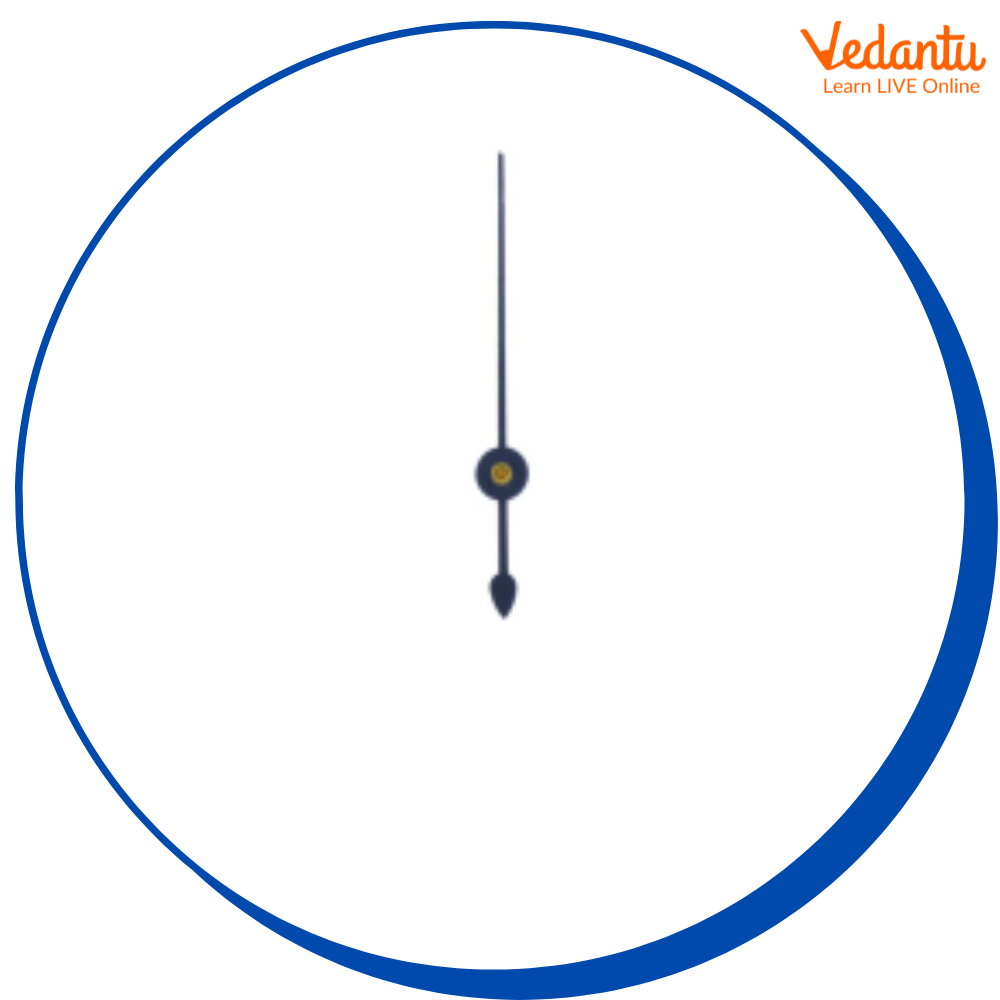Image: Second Hand

## How Does Hour Hand, Minute Hand, and Second Hand Rotate in Analogue Clocks?

### Hour Hand Rotation

The hour hand completes one rotation in 12 hours or two rotations in 24 hours.

### Minute Hand Rotation

The minute hand completes one rotation in 60 minutes.

### Second Hand Rotation

The second hand completes one rotation in one minute.

## How to Read Time in Analogue Clocks?

To read a time using an analogue clock, just look at the hands where they are pointing. The smaller hand, also known as the hour hand, will tell you the hour. The larger hand, also known as the minute hand, will tell you the minutes of the current hour. The thinnest hand, also known as the second hand, will tell you the seconds of the current minute.

Let’s learn to read the time.

Step 1: Look at the hour hand first. This will directly point to the number of hours, or somewhere between that number and the next number.

Example

Here, the hour hand is directly pointed at 10. Hence, the hour is 10.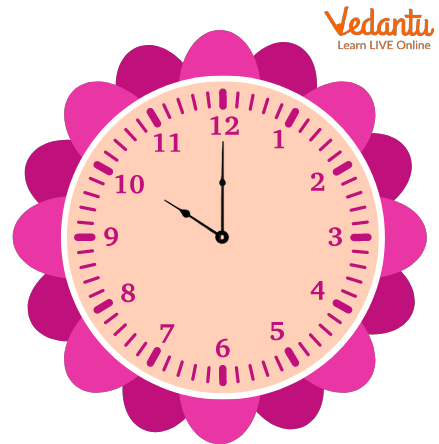Image: An analogue clock showing the 10th hour

Here, the hour hand is between 1 and 2. Hence, the hour is 1.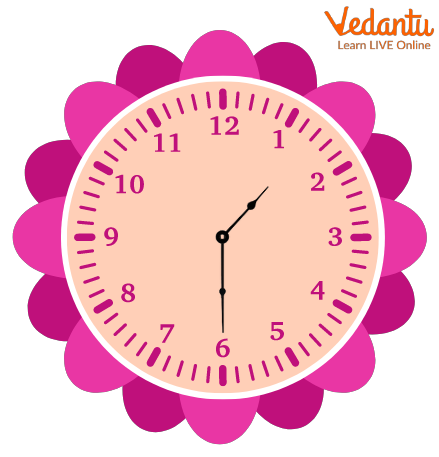Image: An analogue clock showing time as 1:30

Step 2: Now, look at the minute hand. It starts at the top of the clock. For every minute that passes, it moves one tick around the clock.

Now, start from the top and count the tick marks to see how many minutes have passed.

You can count by 1’s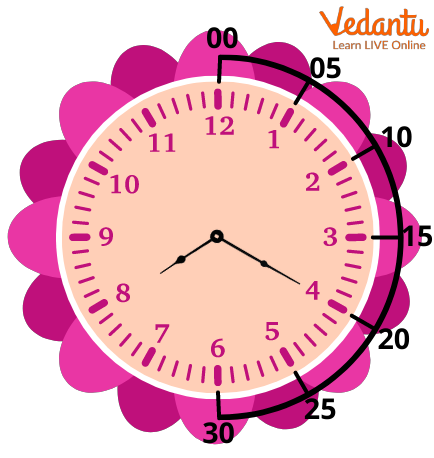Image: An analogue clock showing 8:20
You can count by 5’sImage: An analogue clock showing 8:20

The minute hand in the above clock shows 20 minutes. Also, it is showing 8 hours as the hour hand is between 8 and 9. Therefore, the time is 20 past 8 or 8: 20.

Let’s take one more example to understand in a better way.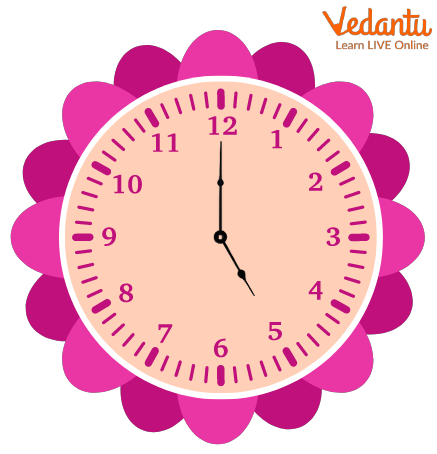Image: An analogue clock showing time as 5

Here, the hour hand is at 5. Hence, the hour is 5.

The minute hand is pointing at 12. This implies 0 minutes after the hour. Therefore, the time is 5:00, or you can also say 5’0 clock.

Step 3: Now, look at the second hand. It works similarly as minute hands. It also starts at the top of the clock. For every second that passes, it moves one tick around the clock. Let us understand with an example: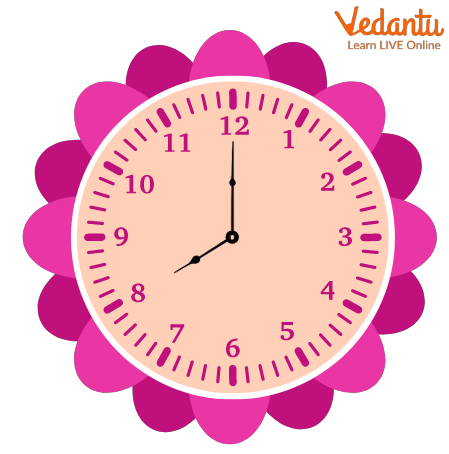Image: An analogue clock showing time as 8

Here, the hour hand is pointed at 8. Hence, the hour is 8.

The minute hand is pointed at 12. This implies 0 minutes after the hour.

The second hand is at 4. This implies that 20 seconds have passed. The time here is 8 hours 0 minutes and 20 seconds.

## Conclusion

In short, an analogue clock is a clock with three moving hands, namely the hour hand, minute hand, and second hand to show you the time. Generally, in an analogue clock, the hour hand is marked from 1 to 12. Also, an analogue clock is named as analogue because it moves smoothly from one point to another without any sudden break or jumps.

Last updated date: 02nd Oct 2023
Total views: 138.3k
Views today: 4.38k

## FAQs on Analogue Clock for Kids to Learn Time

1. What are AM and PM in time?

AM, also known as Anti-Meridian, and PM, also known as Prime Meridian, are used in the 12-hour clock system to differentiate day and night, respectively.  The first 12-hour duration, designated as AM, runs from midnight to noon (12:00 AM to 12:00 PM). The second 12-hour duration, designated as PM, runs from noon to midnight (12:00 PM to 12:00 AM). Therefore, the numbers from 1 to 12 followed by AM or PM are used to identify all 24 hours of the 12-hour clock system.

2. How are minutes and seconds calculated in the analogue clock?

The minutes and seconds in the analogue clock are calculated by multiplying the number by 5. For example, if the minute hand points at 1, then it says 5 minutes have passed. If it points at 2, then it says 10 minutes have passed, and so on.

Similarly, if the second hand points at 1, then it says 5 seconds have passed. If it points at 2, then it says 10 seconds have passed, and so on.